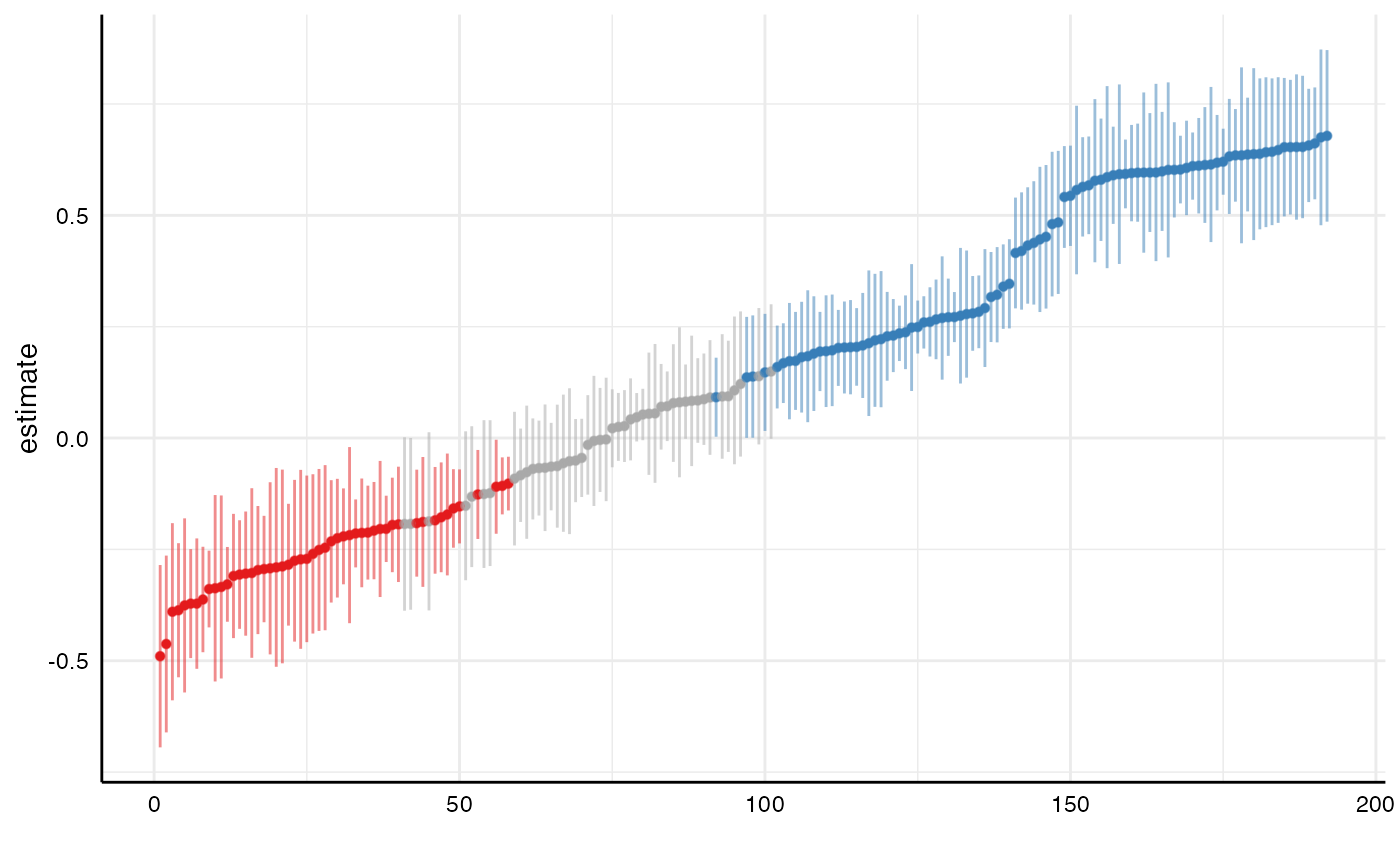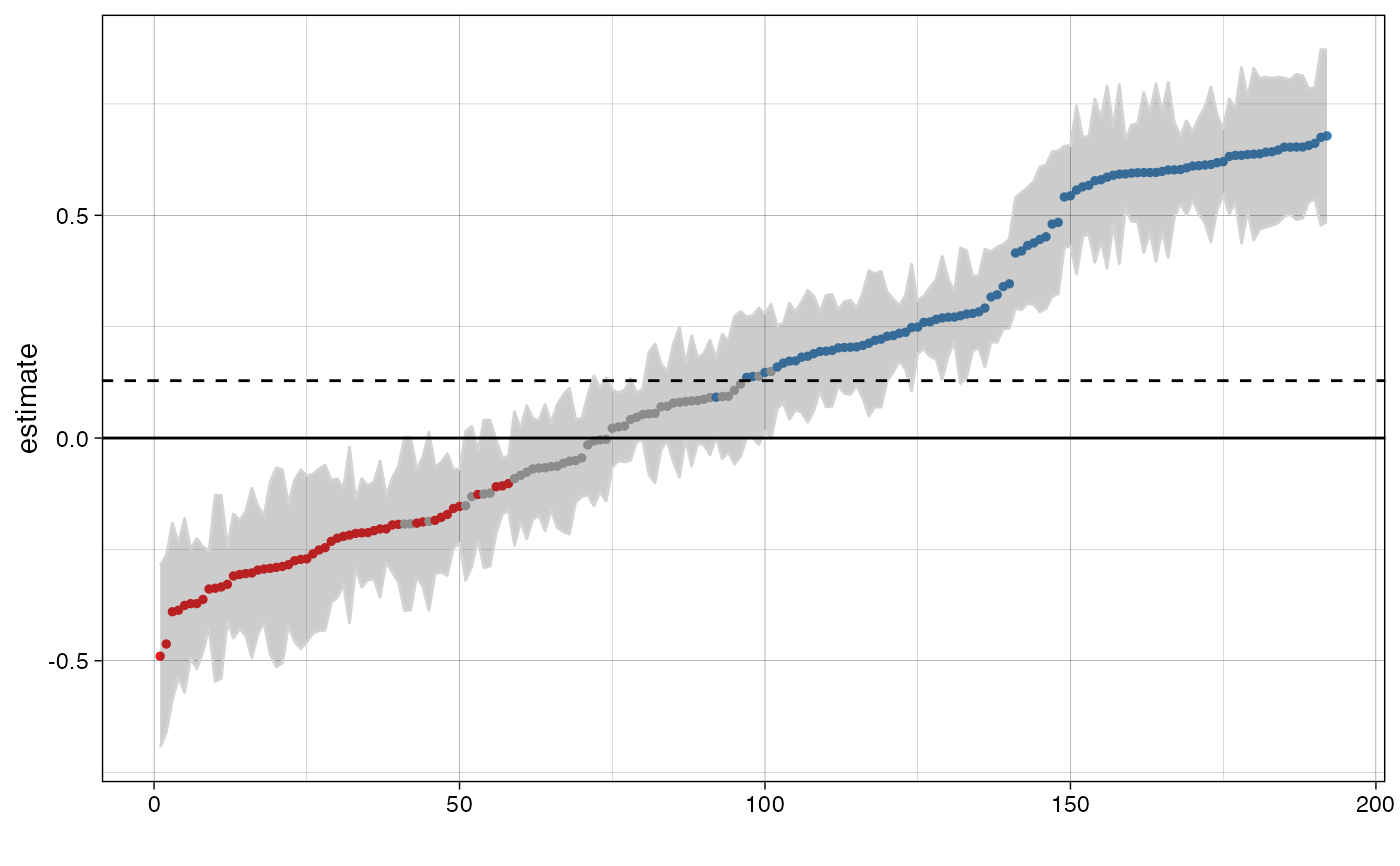This function plots the a ranked specification curve. Confidence intervals can be included. Significant results are highlighted (negative = red, positive = blue, grey = nonsignificant). This functions creates the upper panel in plot_specs().

plot_curve(
df,
var = .data$estimate, desc = FALSE, ci = TRUE, ribbon = FALSE, legend = FALSE, null = 0 ) ## Arguments df a data frame resulting from run_specs(). var which variable should be evaluated? Defaults to estimate (the effect sizes computed by run_specs()). desc logical value indicating whether the curve should the arranged in a descending order. Defaults to FALSE. ci logical value indicating whether confidence intervals should be plotted. ribbon logical value indicating whether a ribbon instead should be plotted. legend logical value indicating whether the legend should be plotted Defaults to FALSE. null Indicate what value represents the null hypothesis (Defaults to zero) ## Value a ggplot object. ## Examples # load additional library library(ggplot2) # for further customization of the plots # Run specification curve analysis results <- run_specs(df = example_data, y = c("y1", "y2"), x = c("x1", "x2"), model = c("lm"), controls = c("c1", "c2"), subsets = list(group1 = unique(example_data$group1),
group2 = unique(example_data$group2))) # Plot simple specification curve plot_curve(results)# Ribbon instead of CIs and customize further plot_curve(results, ci = FALSE, ribbon = TRUE) + geom_hline(yintercept = 0) + geom_hline(yintercept = median(results$estimate),
linetype = "dashed") +
theme_linedraw()